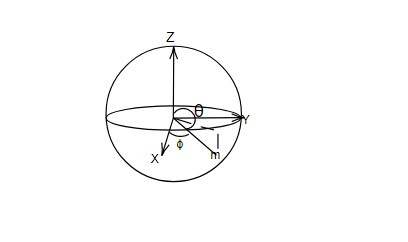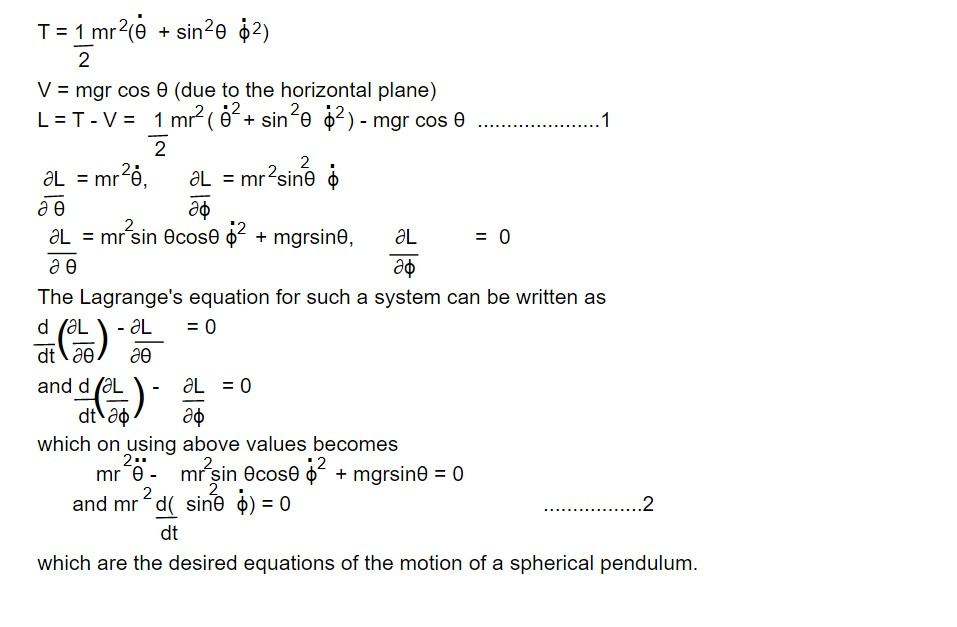# Equation of motion of a spherical pendulum using Lagrange's equation of motion.

A pendulum in which bob is constrained to move on a sphere rather than on a circle is called spherical pendulum. The position of the bob is located by spherical coordinates θ, Ø as shown in fig. The distance r of the bob from the origin will be the radius of the sphere on which the particle moves. The force in this case will not be central, but is constant in the vertical direction.This note is taken from Classical mechanics, MSC physics, Nepal.